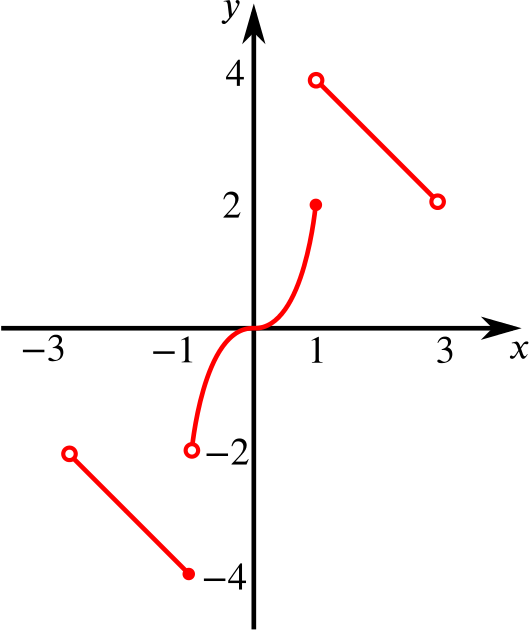Review question

# Can we find the inverse of a function in three parts? Add to your resource collection Remove from your resource collection Add notes to this resource View your notes for this resource

Ref: R8028

## Solution

The function $f$ has domain $\{x: -3< x <3, x \text{ is real}\}$ and is defined by \begin{align*} f:x\to -5-x, \qquad &(-3< x \le -1) \\ f: x\to 2x^3, \qquad&(-1< x \le 1) \\ f:x\to 5-x, \qquad &(1< x <3). \end{align*}

State the range of the function and sketch the graph of $y=f(x)$.

We know that the graph $y=-5-x$ is a straight line on the given interval, running from (-3, -2) down to $(-1,-4)$. Note that $(-1,-4)$ is included in the graph, but $(-3,-2)$ is not.

Similarly, we know that the graph $y=5-x$ is a straight line on the given interval, running from $(1,4)$ down to $(3,2)$. Note that neither $(1,4)$ nor $(3,2)$ is included in the graph.

We should know the graph of $y = x^3$, and $y = 2x^3$ is a stretch of this by a scale factor of $2$ in the $y$-direction. Its starting point on the given interval is $(-1,-2)$, and its endpoint is $(1,2)$. Note that $(1,2)$ is included in the graph, but $(-1,-2)$ is not.

The graph of $y=f(x)$ isA sketch of $y=f(x)$

The solid endpoints are included in the graph, while the empty endpoints are not. Therefore the range of $f$ is $\{y:-4\le y<4, y\ne -2\}$.

Define the inverse relation in similar form and determine whether this inverse relation is a function.

We have that $g$ is given by \begin{align*} g:x\to -5-x, \qquad &(-4\le x<-2) \\ g:x\to (x/2)^{1/3}, \qquad &(-2< x \le2) \\ g:x\to 5-x. \qquad &(2< x <4) \end{align*}

Then $g$ IS a function because, as we can see from our sketch, the function $f$ is $1-1$. The evidence for this is that no horizontal line cuts our graph of $y = f(x)$ in two places.

We say the original function $f$ is injective; no point is mapped to two different points by $g$, the inverse.